• /*二项分布即重复n次的伯努利试验,每次发生的概率一样，假设成功的概率是p，那么失败的概率就是1-p； 二项分布概率公式：试验中发生K次的概率是 P(ξ=K)= C(n,k) * p^k * (1-p)^(n-k), 其中C(n, k) = n!/(k! * (n-...
/*二项分布即重复n次的伯努利试验,每次发生的概率一样，假设成功的概率是p，那么失败的概率就是1-p；
二项分布的概率公式：试验中发生K次的概率是
P(ξ=K)= C(n,k) * p^k * (1-p)^(n-k), 其中C(n, k) = n!/(k! * (n-k)!)
因为这里的数据，k最大为100,100的阶乘超过了int64位，所以不能用这个公式，这时候可以用泊松分布的概率
公式求近似值。
好了接着解释题意：这道题输入n和m，求m是期望，求实验中发生不超过n次的概率，所有所有的概率为0,1,2,3,4...n的概率和
*/
#include<stdio.h>
#include<string.h>
#include<math.h>
#define e exp(1.0)
int main()
{int i,j,n,m,k;int t;double ok;scanf("%d",&t);while(t--){
scanf("%d%lf",&n,&ok);
double num=1.0/( pow(e,ok) );
double sum=0;
for(i=0;i<=n;i++)
{
double f=pow(ok,i)*num;
for(j=1;j<=i;j++)
f=f/j;
sum=sum+f;
}
printf("%.3lf\n",sum);}
return 0;}
lyh走之前还送了一次助攻

展开全文• 二项分布就是独立事件n重伯努利试验，每次试验只有A发生与不发生两种结果，n次试验中恰好发生k次的概率。 P{X=k} = $C_{n}^{k}p^{k}q^{n-k},$k=0,1,2,..n q=1-p; 简记为 X~B(n,p) [二项分布.问题1]：大家知道...
二项分布最大值，泊松分布的推导，几何分布的推导 (概统2.应用)

1.二项分布

二项分布就是独立事件n重伯努利试验，每次试验只有A发生与不发生两种结果，求n次试验中恰好发生k次的概率。

P{X=k} = Cknpkqn−k,${C}_{n}^{k}{p}^{k}{q}^{n-k},$$C_{n}^{k}p^{k}q^{n-k},$k=0,1,2,..n
q=1-p;

简记为 X~B(n,p)

[二项分布.问题1]：大家知道，二项分布中，当N趋于很大，p趋于0时，二项分布可以近似看作泊松分布，可以用泊松分布公式代替二项分布公式计算，泊松分布公式比二项分布公式简单。问，射击例子中，连续射n次，如果n很大，那么射中次数为k次的概率P{X=k}可以用泊松分布吗？

答：这主要看每次射中概率p，泊松分布的条件是n很大，p很小，n趋于无穷，p趋于0，n*p是一个常数λ$\lambda$$\lambda$，（这个常数是事件出现的频率或者总体平均值）；如果射击手每次射击的射中概率p为零点几之类，是不可以用泊松公式计算的，因为泊松公式需要定义一个常数λ$\lambda$$\lambda$ ，这个常数它要么是单位时间内的发生次数，要么是一个集合个体总数N乘以每个个体发生的极小概率p，λ$\lambda$$\lambda$=N*p；在射击例子中，射中概率p并不是很小很小，无法定义单位时间内射中次数，也无法定义N，所以不能用泊松分布公式计算。必须用标准的二项公式计算。
所以说，射击n次，射中k次的概率，是典型的二项分布的实例。

[二项分布.问题2： k取多少时，二项分布P{X=k}达到最大值？]
二项分布的公式是
P{X=k} = Cknpkqn−k,${C}_{n}^{k}{p}^{k}{q}^{n-k},$$C_{n}^{k}p^{k}q^{n-k},$k=0,1,2,..n, q=1-p;
记为 X~B(n,p)
k等于多少时，P{X=k}达到最大值？具有最大值？

可以采用比值法，因为二项分布的P(X)分布律的函数趋势与泊松分布，与正态分布类似，都是先逐渐向上，再逐渐向下，中间有一个最大值。
【二项分布，n很大，p趋于0时，演变成泊松分布；泊松分布，当随机变量取连续值时，演变成正态分布】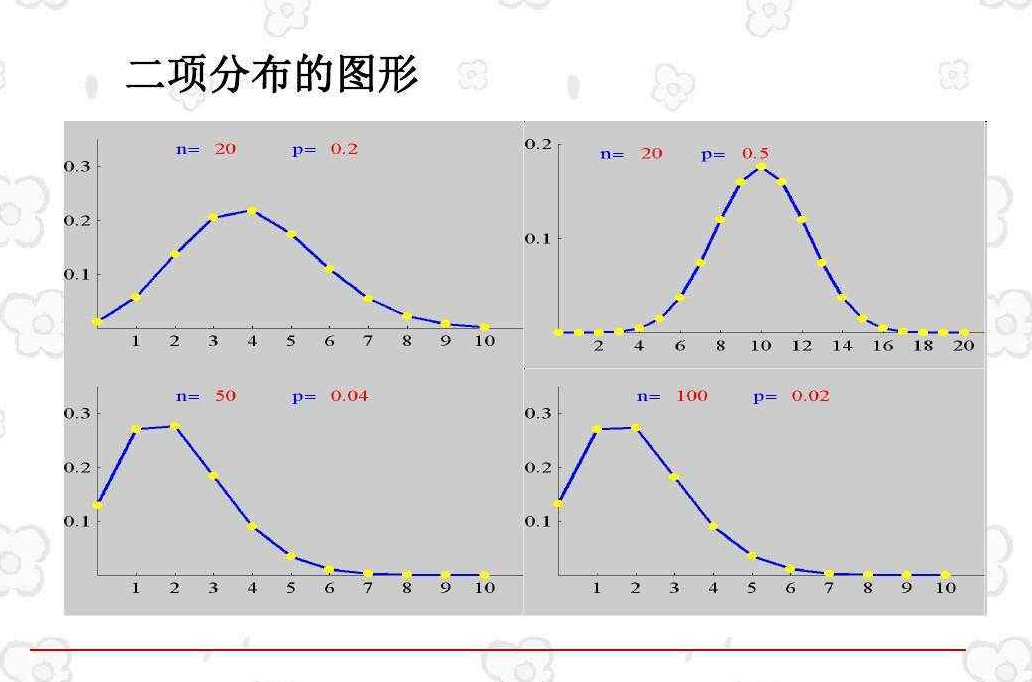图形在最大值前单调增，最大值后单调减
可以采用比值法，但是有两个比值
k>=0 , P(X=(k+1))P(X=k)$\frac{P\left(X=\left(k+1\right)\right)}{P\left(X=k\right)}$$\frac{P(X=(k+1))}{P(X=k)}$ 由 大于1（单调增），转为小于1（单调减），等于1时，k最大

k>=1 , P(X=k)P(X=(k−1))$\frac{P\left(X=k\right)}{P\left(X=\left(k-1\right)\right)}$$\frac{P(X=k)}{P(X=(k-1))}$ 由 大于1（单调增），转为小于1（单调减），等于1时，k最大

计算，P(X=(k+1))P(X=k)$\frac{P\left(X=\left(k+1\right)\right)}{P\left(X=k\right)}$$\frac{P(X=(k+1))}{P(X=k)}$ >=1  ；(1)，k的值

再计算，P(X=k)P(X=(k−1))$\frac{P\left(X=k\right)}{P\left(X=\left(k-1\right)\right)}$$\frac{P(X=k)}{P(X=(k-1))}$ >=1  ；(2)，k的值

设 q=1-p;

展开计算(1)式
P(X=(k+1))P(X=k)$\frac{P\left(X=\left(k+1\right)\right)}{P\left(X=k\right)}$$\frac{P(X=(k+1))}{P(X=k)}$ = Ck+1npk+1qn−k−1Cknpkqn−k$\frac{{C}_{n}^{k+1}{p}^{k+1}{q}^{n-k-1}}{{C}_{n}^{k}{p}^{k}{q}^{n-k}}$$\frac{C_{n}^{k+1}p^{k+1}q^{n-k-1}}{C_{n}^kp^kq^{n-k}}$ = Ck+1nCkn∗pq$\frac{{C}_{n}^{k+1}}{{C}_{n}^{k}}\ast \frac{p}{q}$$\frac{C_{n}^{k+1}}{C_{n}^{k}}*\frac{p}{q}$ =n!(n−k−1)!(k+1)!n!(n−k)!k!p1−p$\frac{\frac{n!}{\left(n-k-1\right)!\left(k+1\right)!}}{\frac{n!}{\left(n-k\right)!k!}}\frac{p}{1-p}$$\frac{\frac{n!}{(n-k-1)!(k+1)!}}{\frac{n!}{(n-k)!k!}}\frac{p}{1-p}$
=(n−k)!k!(n−k−1)!(k+1)!∗p1−p$\frac{\left(n-k\right)!k!}{\left(n-k-1\right)!\left(k+1\right)!}\ast \frac{p}{1-p}$$\frac{(n-k)!k!}{(n-k-1)!(k+1)!}*\frac{p}{1-p}$ =n−kk+1∗p1−p$\frac{n-k}{k+1}\ast \frac{p}{1-p}$$\frac{n-k}{k+1}*\frac{p}{1-p}$ >=1
得到
(n-k)p >= (k+1)(1-p)
np-kp >= k+1 -kp -p
最后得到
k<=(n+1)p -1   ；(3)

展开计算(2)式
P(X=k)P(X=(k−1))$\frac{P\left(X=k\right)}{P\left(X=\left(k-1\right)\right)}$$\frac{P(X=k)}{P(X=(k-1))}$=Cknpkqn−kCk−1npk−1qn−k+1$\frac{{C}_{n}^{k}{p}^{k}{q}^{n-k}}{{C}_{n}^{k-1}{p}^{k-1}{q}^{n-k+1}}$$\frac{C_{n}^{k}p^kq^{n-k}}{C_{n}^{k-1}p^{k-1}q^{n-k+1}}$=CknCk−1n∗pq$\frac{{C}_{n}^{k}}{{C}_{n}^{k-1}}\ast \frac{p}{q}$$\frac{C_{n}^{k}}{C_{n}^{k-1}}*\frac{p}{q}$=n!(n−k)!k!n!(n−k+1)!(k−1)!p1−p$\frac{\frac{n!}{\left(n-k\right)!k!}}{\frac{n!}{\left(n-k+1\right)!\left(k-1\right)!}}\frac{p}{1-p}$$\frac{\frac{n!}{(n-k)!k!}}{\frac{n!}{(n-k+1)!(k-1)!}}\frac{p}{1-p}$
= (n−k+1)!(k−1)!(n−k)!k!∗p1−p$\frac{\left(n-k+1\right)!\left(k-1\right)!}{\left(n-k\right)!k!}\ast \frac{p}{1-p}$$\frac{(n-k+1)!(k-1)!}{(n-k)!k!}*\frac{p}{1-p}$ = n−k+1k∗p1−p$\frac{n-k+1}{k}\ast \frac{p}{1-p}$$\frac{n-k+1}k*\frac{p}{1-p}$ >=1
得到
(n-k+1)p >= k(1-p)
np-kp+p >= k-kp
最后得到
k<=(n+1)p   ；(4)
==
k<=(n+1)p -1  ； (3)
k<=(n+1)p       ；(4)
结合(3)和(4)，
当 (n+1)p 刚好为整数时（没有余数，没有小数点后面）
k的最大值有两个，分别是
k1=(n+1)p -1
k2=(n+1)p
当 (n+1)p 不为整数时（有余数，有小数点后面的数），只有一个最大值，
取(4)的整数部分（向下取整） ，floor((n+1)p); 向下取整数部分
k= floor( (n+1)p ) = (n+1)p ;

2. 二项分布演变到泊松分布的推导

大家知道二项分布当n很大，p很小，p趋于0时，即n→∞,p→0$n\to \mathrm{\infty },p\to 0$$n\to\infty, p\to0$ , np= λ$\lambda$$\lambda$ ，λ$\lambda$$\lambda$ 是一个常数的情况下，可以用泊松分布近似代替二项分布。那么怎样推导呢？下面是推导过程**

泊松分布的公式：
P{X=k}   = (λt)kk!∗e−(λt)$\frac{\left(\lambda t{\right)}^{k}}{k!}\ast {e}^{-\left(\lambda t\right)}$$\frac{(\lambda t)^k}{ k!}* e^{- (\lambda t)}$  , (k=0,1,2,…)
当取单位时间 t=1时，泊松分布的公式简化为：
P{X=k}   = λkk!∗e−λ$\frac{{\lambda }^{k}}{k!}\ast {e}^{-\lambda }$$\frac{\lambda ^k}{ k!}* e^{- \lambda }$  , (k=0,1,2,…)

简记为：X~P(λ)$P\left(\lambda \right)$$P(\lambda )$

由二项分布的公式得，
P{X=k} = Cknpkqn−k,${C}_{n}^{k}{p}^{k}{q}^{n-k},$$C_{n}^{k}p^{k}q^{n-k},$k=0,1,2,..n
其中，q=1-p;

当，limt→−∞，p→0$\underset{t\to -\mathrm{\infty }，p\to 0}{lim}$$\lim_{t\to-\infty ，p\to0}$，时
P{X=k} = Cknpk(1−p)n−k${C}_{n}^{k}{p}^{k}\left(1-p{\right)}^{n-k}$$C_{n}^{k}p^{k}(1-p)^{n-k}$
= n!(n−k)!∗k!∗pk∗(1−p)n−k$\frac{n!}{\left(n-k\right)!\ast k!}\ast {p}^{k}\ast \left(1-p{\right)}^{n-k}$$\frac{n!}{(n-k)!* k!}*p^k*(1-p)^{n-k}$

=n∗(n−1)∗(n−2)∗...(n−(k−1))(n−k)!(n−k)!∗k!∗pk∗(1−p)n−k$\frac{n\ast \left(n-1\right)\ast \left(n-2\right)\ast ...\left(n-\left(k-1\right)\right)\left(n-k\right)!}{\left(n-k\right)!\ast k!}\ast {p}^{k}\ast \left(1-p{\right)}^{n-k}$$\frac{n*(n-1)*(n-2)*...(n-(k-1))(n-k)!}{(n-k)!* k!}*p^k*(1-p)^{n-k}$

=n∗(n−1)∗(n−2)∗...(n−(k−1))k!∗pk∗(1−p)n−k$\frac{n\ast \left(n-1\right)\ast \left(n-2\right)\ast ...\left(n-\left(k-1\right)\right)}{k!}\ast {p}^{k}\ast \left(1-p{\right)}^{n-k}$$\frac{n*(n-1)*(n-2)*...(n-(k-1))}{ k!}*p^k*(1-p)^{n-k}$    (1)

注：对于 n∗(n−1)∗(n−2)∗...(n−(k−1))$n\ast \left(n-1\right)\ast \left(n-2\right)\ast ...\left(n-\left(k-1\right)\right)$$n*(n-1)*(n-2)*...(n-(k-1))$ 总共有k项（从(n-0) 到 (n-(k-1)) )
当 n→∞$n\to \mathrm{\infty }$$n\to\infty$时，k是一个有限数（n是总样本数，k是发生故障的次数），k与n相加减，k可以忽略掉，
limn−>∞(n∗(n−1)∗(n−2)∗...(n−(k−1)))≈$\underset{n->\mathrm{\infty }}{lim}\left(n\ast \left(n-1\right)\ast \left(n-2\right)\ast ...\left(n-\left(k-1\right)\right)\right)\approx$$\lim_{n->\infty } (n*(n-1)*(n-2)*...(n-(k-1))) \approx$ nk${n}^{k}$$n^k$ （k被忽略掉了）
同理：
limn−>∞(1−p)n−k≈$\underset{n->\mathrm{\infty }}{lim}\left(1-p{\right)}^{n-k}\approx$$\lim_{n->\infty } (1-p)^{n-k} \approx$ (1−p)n$\left(1-p{\right)}^{n}$$(1-p)^n$  （k被忽略掉了）

接上面（1）式，
当，limt→−∞，p→0$\underset{t\to -\mathrm{\infty }，p\to 0}{lim}$$\lim_{t\to-\infty ，p\to0}$，时
（1）式等于，
P{X=k} =n∗(n−1)∗(n−2)∗...(n−(k−1))k!∗pk∗(1−p)n−k$\frac{n\ast \left(n-1\right)\ast \left(n-2\right)\ast ...\left(n-\left(k-1\right)\right)}{k!}\ast {p}^{k}\ast \left(1-p{\right)}^{n-k}$$\frac{n*(n-1)*(n-2)*...(n-(k-1))}{ k!}*p^k*(1-p)^{n-k}$

=nkk!∗pk∗(1−p)n−k$=\frac{{n}^{k}}{k!}\ast {p}^{k}\ast \left(1-p{\right)}^{n-k}$$=\frac{n^k}{ k!}*p^k*(1-p)^{n-k}$

=nkk!∗pk∗(1−p)n$=\frac{{n}^{k}}{k!}\ast {p}^{k}\ast \left(1-p{\right)}^{n}$$=\frac{n^k}{ k!}*p^k*(1-p)^{n}$   (2)

又注：n*p 意味着单位时间内发生的次数，定义为一个常数λ$\lambda$$\lambda$,
λ$\lambda$$\lambda$=n*p ， n=λp$n=\frac{\lambda }{p}$$n = \frac{\lambda}{p}$

接上面（2）式就等于，
当，limt→−∞，p→0$\underset{t\to -\mathrm{\infty }，p\to 0}{lim}$$\lim_{t\to-\infty ，p\to0}$，时
P{X=k}  =nkk!∗pk∗(1−p)n$\frac{{n}^{k}}{k!}\ast {p}^{k}\ast \left(1-p{\right)}^{n}$$\frac{n^k}{ k!}*p^k*(1-p)^{n}$

= (np)kk!∗(1−p)n$\frac{\left(np{\right)}^{k}}{k!}\ast \left(1-p{\right)}^{n}$$\frac{(np)^k}{ k!}*(1-p)^{n}$

= λkk!∗(1−p)n$\frac{{\lambda }^{k}}{k!}\ast \left(1-p{\right)}^{n}$$\frac{\lambda^k}{ k!}*(1-p)^{n}$

= λkk!∗(1−p)λp$\frac{{\lambda }^{k}}{k!}\ast \left(1-p{\right)}^{\frac{\lambda }{p}}$$\frac{\lambda^k}{ k!}*(1-p)^{\frac{\lambda}{p}}$    (3)

又注：设 t=−1p$t=-\frac{1}{p}$$t = - \frac{1}{p}$ ,
那么 ， p=−1t,(1−p)=(1+1t)$p=-\frac{1}{t},\left(1-p\right)=\left(1+\frac{1}{t}\right)$$p = - \frac{1}{t}, (1-p) = (1+\frac{1}{t})$
λp$\frac{\lambda }{p}$$\frac{\lambda}{p}$ = -λt$\lambda t$$\lambda t$ =t(−λ)$t\left(-\lambda \right)$$t (-\lambda)$
(1−p)λp=(1+1t)t(−λ)$\left(1-p{\right)}^{\frac{\lambda }{p}}=\left(1+\frac{1}{t}{\right)}^{t\left(-\lambda \right)}$$(1-p)^{\frac{\lambda}{p}} =(1+\frac{1}{t})^{t(-\lambda)}$
因为 p→0$p\to 0$$p \to 0$, 所以，t→−∞$t\to -\mathrm{\infty }$$t \to -\infty$ , −t→∞$-t\to \mathrm{\infty }$$-t \to \infty$

那么，上面（3）式就得到：
当，limt→−∞，p→0$\underset{t\to -\mathrm{\infty }，p\to 0}{lim}$$\lim_{t\to-\infty ，p\to0}$，时
P{X=k} = λkk!∗(1−p)λp$\frac{{\lambda }^{k}}{k!}\ast \left(1-p{\right)}^{\frac{\lambda }{p}}$$\frac{\lambda^k}{ k!}*(1-p)^{\frac{\lambda}{p}}$
= λkk!∗(1+1t)t(−λ)$\frac{{\lambda }^{k}}{k!}\ast \left(1+\frac{1}{t}{\right)}^{t\left(-\lambda \right)}$$\frac{\lambda^k}{ k!}*(1+\frac{1}{t})^{t (-\lambda) }$    (4)

又注： 由e的定义式（e的公式）得：
每次加无限小分份量1n$\frac{1}{n}$$\frac{1}{n}$，再累积无限多次{n}，就是e
参考：对自然数e的理解，e的证明，e的计算（基础）
e=limn−>∞(1+1n)n$\underset{n->\mathrm{\infty }}{lim}\left(1+\frac{1}{n}{\right)}^{n}$$\lim_{n->\infty }(1+\frac{1}{n})^{n }$
e=limn−>−∞(1+1n)n$\underset{n->-\mathrm{\infty }}{lim}\left(1+\frac{1}{n}{\right)}^{n}$$\lim_{n->-\infty }(1+\frac{1}{n})^{n }$
在那篇博文有证明得出，n无论趋于正无穷，还是趋于负无穷，(1+1n)n$\left(1+\frac{1}{n}{\right)}^{n}$$(1+\frac{1}{n})^n$结果都是e
因此得：limt→−∞(1+1t)t=e$\underset{t\to -\mathrm{\infty }}{lim}\left(1+\frac{1}{t}{\right)}^{t}=e$$\lim_{t\to-\infty }(1+\frac{1}{t})^{t}=e$
====

n→−∞$n\to -\mathrm{\infty }$$n\to-\infty$ 的证明方法：
设n=1x$n=\frac{1}{x}$$n=\frac{1}{x}$， 利用 limx→0(1+x)1n=e$\underset{x\to 0}{lim}\left(1+x{\right)}^{\frac{1}{n}}=e$$\lim_{x\to0}(1+x)^{\frac{1}{n}} = e$;
===
直观理解，如果t趋于负无穷，那么指数(t)就是累积除的关系，
每次减无限小分份量1t$\frac{1}{t}$$\frac{1}{t}$，但是累积除以无限多次{t}，还是等于e
limt→−∞(1+1t)t=e$\underset{t\to -\mathrm{\infty }}{lim}\left(1+\frac{1}{t}{\right)}^{t}=e$$\lim_{t\to-\infty }(1+\frac{1}{t})^{t}=e$

那么，上面（4）式就得到：
当，limt→−∞，p→0$\underset{t\to -\mathrm{\infty }，p\to 0}{lim}$$\lim_{t\to-\infty ，p\to0}$，时
P{X=k}   = λkk!∗(1+1t)t(−λ)$\frac{{\lambda }^{k}}{k!}\ast \left(1+\frac{1}{t}{\right)}^{t\left(-\lambda \right)}$$\frac{\lambda^k}{ k!}*(1+\frac{1}{t})^{t (-\lambda )}$

= λkk!∗(e)−λ$\frac{{\lambda }^{k}}{k!}\ast \left(e{\right)}^{-\lambda }$$\frac{\lambda^k}{ k!}* (e)^{- \lambda }$  = λkk!∗e−λ$\frac{{\lambda }^{k}}{k!}\ast {e}^{-\lambda }$$\frac{\lambda^k}{ k!}* e^{- \lambda }$

P{X=k}   = λkk!∗e−λ$\frac{{\lambda }^{k}}{k!}\ast {e}^{-\lambda }$$\frac{\lambda^k}{ k!}* e^{- \lambda }$  , (k=0,1,2,…)

如果用单位时间内发生次数来表达，λ$\lambda$$\lambda$ 可以表示成 λt$\lambda t$$\lambda t$
上式可以表示成：
当，limt→−∞，p→0$\underset{t\to -\mathrm{\infty }，p\to 0}{lim}$$\lim_{t\to-\infty ，p\to0}$，时
P{X=k}   = (λt)kk!∗e−(λt)$\frac{\left(\lambda t{\right)}^{k}}{k!}\ast {e}^{-\left(\lambda t\right)}$$\frac{(\lambda t)^k}{ k!}* e^{- (\lambda t)}$  , (k=0,1,2,…)

3.泊松分布公式与 自然数 的定义 e

参考前面博文对自然数e的理解，证明，计算(基础)

ex${e}^{x}$$e^{x}$ = ∑∞i=0(x)ii!$\sum _{i=0}^{\mathrm{\infty }}\frac{\left(x{\right)}^{i}}{i!}$$\sum_{i=0}^{\infty}\frac{(x)^{i}}{i!}$
ex${e}^{x}$$e^{x}$ = 1+x+(x)22!+(x)33!+...+(x)n1n!$\frac{\left(x{\right)}^{2}}{2!}+\frac{\left(x{\right)}^{3}}{3!}+...+\left(x{\right)}^{n}\frac{1}{n!}$$\frac{(x)^2}{2!}+\frac{(x)^3}{3!} + ... +(x)^{n}\frac{1}{n!}$
===
近似计算（e<4）的情况，e的指数越大，后面的项越大，越需要多项展开）：
ex${e}^{x}$$e^{x}$ = 1+x+(x)22+(x)36+(x)424+(x)5120$\frac{\left(x{\right)}^{2}}{2}+\frac{\left(x{\right)}^{3}}{6}+\frac{\left(x{\right)}^{4}}{24}+\frac{\left(x{\right)}^{5}}{120}$$\frac{(x)^2}{2}+\frac{(x)^3}{6} + \frac{(x)^4}{24} +\frac{(x)^5}{120}$

=====
思考问题：
ex${e}^{x}$$e^{x}$的泰勒级数展开多项式中，哪一项的值最大？
答案是：x等于多少就是哪项值最大，恰好第x项的值最大。
可以参考前面博文对自然数e的理解，证明，计算(基础)
物理意义：单位时间内事件发生的次数最大可能性就是平均概率。
===
比如x=1，第一项最大 x=1。
x=2:  第一项及第二项 都是最大(x)22=222=2$\frac{\left(x{\right)}^{2}}{2}=\frac{{2}^{2}}{2}=2$$\frac{(x)^2}{2}=\frac{2^2}{2}=2$；
x=3，第三项 最大(x)36=336$\frac{\left(x{\right)}^{3}}{6}=\frac{{3}^{3}}{6}$$\frac{(x)^3}{6}=\frac{3^3}{6}$=4.73；
x=4，第四项 最大(x)424=4424=25624$\frac{\left(x{\right)}^{4}}{24}=\frac{{4}^{4}}{24}=\frac{256}{24}$$\frac{(x)^4}{24}=\frac{4^4}{24}=\frac{256}{24}$=12；
以此类推….

可以看到 λkk!$\frac{{\lambda }^{k}}{k!}$$\frac{\lambda^{k} }{k!}$ 其实就是 eλ${e}^{\lambda }$$e^{\lambda}$ 的第k项。
也就是等于说，P{X=k}的概率，就是eλ${e}^{\lambda }$$e^{\lambda}$ 的第k项 的占比例。

[泊松分布.问题1，泊松分布P{x=k}，k等于多少时，P{X=k}最大？]
已知泊松分布的公式，
P{X=k}   = λkk!∗e−λ$\frac{{\lambda }^{k}}{k!}\ast {e}^{-\lambda }$$\frac{\lambda^k}{ k!}* e^{- \lambda }$  , (k=0,1,2,…)
记为 X~P(λ$\lambda$$\lambda$)

P{X=k}的值，就是eλ${e}^{\lambda }$$e^{\lambda}$ 的第k项 的占比例, 那么 k等于多少时，P{X=k}最大？

从直观上理解，单位时间最有可能发生的次数当然是平均数。
从e的泰勒展开多项式理解，eλ${e}^{\lambda }$$e^{\lambda}$的泰勒展开式，就是第λ$\lambda$$\lambda$项值最大。

也就是 k = λ$\lambda$${\lambda}$ 时， P{X=λ$\lambda$${\lambda}$} 取得最大值。

4.二项分布到几何分布的推导

几何分布也是从二项分布而来。实际背景是重复独立试验下首次成功的概率（n重伯努利试验，首次成功的 n 值）
举例：射击n次，首次射中时的n值。
有放回地抽取样品，首次抽到次品时的抽取次数。

二项分布公式，重复试验n次，成功k次的概率：
P{X=k} = Cknpkqn−k,${C}_{n}^{k}{p}^{k}{q}^{n-k},$$C_{n}^{k}p^{k}q^{n-k},$k=0,1,2,..n

重复试验n次，成功1次的概率：
P{X=1} = C1np(1−p)n−1,${C}_{n}^{1}p\left(1-p{\right)}^{n-1},$$C_{n}^{1}p(1-p)^{n-1},$

成功的这一次，恰好是第一次的概率
从1到n，一共射击了n次，如果射击n次仅成功1次，成功的这一次有可能是是第1次，有可能是第2次，，，有可能是第n次，共有n种可能，
所以，成功的这一次，恰好是第一次的概率 = P(X=1)n$\frac{P\left(X=1\right)}{n}$$\frac{P(X=1)}{n}$ = C1np(1−p)n−1n$\frac{{C}_{n}^{1}p\left(1-p{\right)}^{n-1}}{n}$$\frac{C_{n}^{1}p(1-p)^{n-1}}{n}$  =p(1−p)n−1$p\left(1-p{\right)}^{n-1}$$p(1-p)^{n-1}$

所以，几何分布公式（事件首次发生的n值分布）：

P{X=n} = p(1−p)n−1$p\left(1-p{\right)}^{n-1}$$p(1-p)^{n-1}$ ；

纪为 X~G(p)
展开全文泊松分布 几何分布
• 几何分布、二项分布、泊松分布 几何分布 概率的几何分布公式 q=(1-p) r是为了取得首次成功所需进行的试验次数 几何分布 进行一系列相互独立的试验 单次试验的成功概率相等且不等于1 目的是取得第一次成功需要进行...
几何分布、二项分布、泊松分布
几何分布
概率的几何分布公式
q=(1-p)
r是为了取得首次成功所需进行的试验次数
几何分布
进行一系列相互独立的试验
单次试验的成功概率相等且不等于1
目的是求取得第一次成功需要进行多少次试验
几何分布的情况下，第一次取得成功的概率最大期望计算公式方差计算公式二项分布
二项分布公式
进行一系列独立试验
每次试验都有成功和失败的可能，且每次试验成功的可能相同
试验次数有限写作当p接近0.5时图形对称，小于0.5向右偏，大于0.5向左偏。期望和方差泊松分布
条件：

单独时间在给定区间内随机、独立地发生，区间可以是时间或者空间
已知该区间内事件平均发生次数，且为有限数值，平均次数用λ表示

写作公式
给定区间内发生概率为λ，发生r次事件的概率e是一个数学常数，一般为2.718
期望和方差如果X和Y是独立随机变量则泊松分布在特定条件下可近似代替二项分布
一般为n大于50且p小于0.1展开全文统计学 统计学基础
• 极大似然估计法是点估计的一种方法，最早由高斯提出，后来费歇尔(Fisher)在1912年重新提出。它属于数理统计的范畴。大学期间我们都学过概率论和数理统计这门课程。概率论和数理统计是互逆的过程。概率论可以看成是...
极大似然估计法是求点估计的一种方法，最早由高斯提出，后来费歇尔(Fisher)在1912年重新提出。它属于数理统计的范畴。大学期间我们都学过概率论和数理统计这门课程。概率论和数理统计是互逆的过程。概率论可以看成是由因推果，数理统计则是由果溯因。用两个简单的例子来说明它们之间的区别。由因推果(概率论)例1：设有一枚骰子，2面标记的是“正”，4面标记的是“反”。共投掷10次，问：5次“正”面朝上的概率？解：记 “正面”朝上为事件A，正面朝上的次数为x。由题意可知 :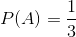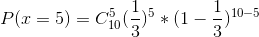更一般的有：例2: 设有一枚骰子，其中“正面”所占的比例为ωω 。共投掷nn 次，问：kk 次“正”面朝上的概率？解：记 “正面”朝上为事件A，正面朝上的次数为x。有题意可知: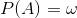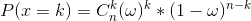例3：设有一枚骰子，做了nn 次实验，其中kk 次“正面”朝上。问：这枚骰子中，“正面”所占的比例ωω 是多少？在例2中，因为我们对骰子模型了解的很透彻，即知道这类实验中ωω 的具体数值。因此可以预测某一事件发生的概率。在例3中，我们并不能完全了解模型精确参数。我们需要通过实验结果来估计模型参数。也就是由果溯因(数理统计)。总结来看如下：例2已知 ω求事件发生的 k次的概率例3已知事件发生了 k次估计 ωPDFGiving ωCalculate the probability distribution of random variableLFGiving random variableCalculate the the probability distribution of ω由于事件发生的概率越大，就越容易发生。所以例3可理解为：ω是多大时，k次“正面”朝上发生的概率最大？计算的时候，对表达式求最大值，得到参数值估计值。这就是极大似然估计方法的原理：用使概率达到最大的那个ω ^ω^ 来估计未知参数ω。这也把一个参数估计问题转化为一个最优化问题。此外，我们甚至不知道一个系统的模型是什么。因此在参数估计前，先按照一定的原则选择系统模型，再估计模型中的参数。本文为了简单，模型设定为伯努利模型。以上是对极大似然估计方法理论上的介绍，接下来介绍计算方法。计算方法为了表述规范，引入概率密度函数：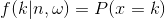通过调换“实验结果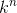”与“模型参数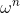”的位置有 似然函数：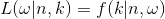通过例4 介绍概率密度函数与似然函数之间的区别：例4.1 设有一枚骰子，1面标记的是“正”，4面标记的是“反”。共投掷10次，设“正面”的次数为k，求k的概率密度函数。解：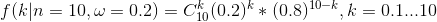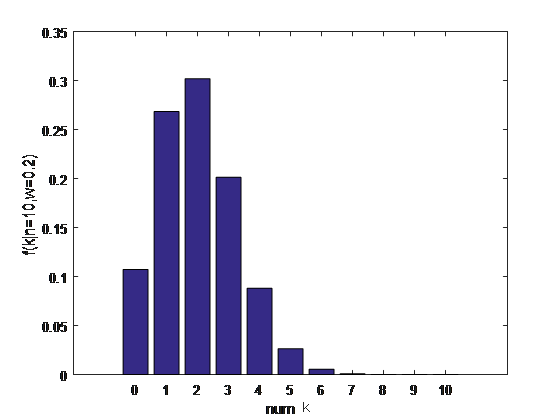从图中可以看出，“正面”次数为2的概率最大。它是关于k的函数。例4.2 设有一枚骰子。共投掷10次，“正面”的次数为2，求“正面”所占的比例，即ω的值。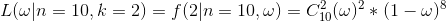似然函数：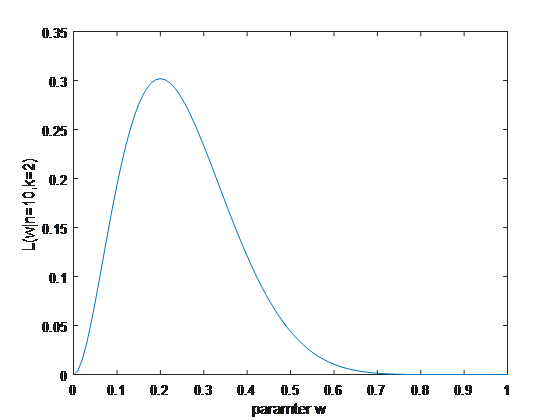因此概率密度函数是指 在参数已知的情况下，随机变量的概率分布情况。似然函数是指 在随机变量已知的情况下，参数取值的概率分布情况。例5：设有一枚骰子，做了10次实验，其中3次“正面”朝上。问：这枚骰子中，“正面”所占的比例是多少？解：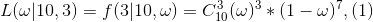我们根据极大似然估计方法的原理：用使概率达到最大的那个ω ̂来估计未知参数ω对于简单的连续函数，求最大值的方法为：函数表达式一阶导数等于0，二阶导数小于0。为了计算简单，对上式两边取对数：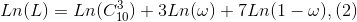一阶条件：将(2)式对ω求偏导数(导数)：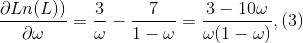令(3)式为0，解得ω=0.3二阶条件：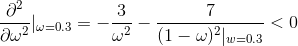因此 ω=0.3时，(1)式取得最大值。根据极大似然估计理论，“正面”所占的比例为0.3例6：设有一枚神奇的骰子，“正面”所占的比例为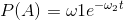。t代表实验时间点。 已知：在t i =1,3,6,9,12,18共6个时刻做实验，每个时刻做n=100次实验。“正面”朝上的次数分别为：x i =94,77,40,26,24,16求：参数ω=(ω 1 ，ω 2 )>0的估计值，。解：求出“正面”朝上的概率密度函数：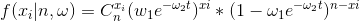似然函数：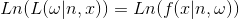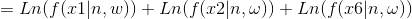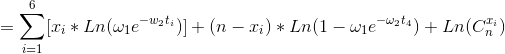对于这样一个复杂的非线性约束优化问题，利用求导的方式不再可行。可借助matlab进行计算。###代码如下：     function f = objfun( x )f = -(94*log(x(1)*exp(-x(2)*1))+6*log(1-(x(1)*exp(-x(2)*1))) + ...77*log(x(1)*exp(-x(2)*3))+23*log(1-(x(1)*exp(-x(2)*3))) + ...40*log(x(1)*exp(-x(2)*6))+60*log(1-(x(1)*exp(-x(2)*6))) + ...26*log(x(1)*exp(-x(2)*9))+74*log(1-(x(1)*exp(-x(2)*9))) + ...24*log(x(1)*exp(-x(2)*12))+76*log(1-(x(1)*exp(-x(2)*12))) + ...16*log(x(1)*exp(-x(2)*18))+84*log(1-(x(1)*exp(-x(2)*18))));endsample5.mx0 = [0.1,0.1];   %给定初值lb = [0,0];     %给定下限ub = [];            %给定上限[x,fval] = fmincon(@objfun,x0,[],[],[],[],lb,ub)123456789101112131415解得：x =1.070111883136768   0.130825782195123fval =3.053055671586732e+02本笔记参考https://blog.csdn.net/chenjianbo88/article/details/52398181https://blog.csdn.net/saltriver/article/details/63681339及李航的《统计学方法》第一章
展开全文• （一）二项分布最大似然估计  二项分布中，有两个结果：事件要么发生，要么不发生。二项分布中，随机变量X取值1表示事件发生，而取值0表示事件不发生。令p表示事件发生的概率，则(1-p)为事件不发生的概率。如...
• 这个多个类别数据虽然是一个概率分布，但数学期望或方差不同，每次取得一个数据时也不知道这个数据是哪个类别下，每个数据属于哪个类别的信息是一个隐含变量，遇到这种情况时我们不能直接用最大似然法。EM算法中文...自然语言处理 深度学习 机器学习
• 二项分布、多项分布和联合概率分布数学表达形式 2.求解步骤 2.1带约束的极值问题，引入拉格朗日乘子 2.2极值，其实就是偏导 2.3后验证概率最大二项分布和多项分布 什么是二项分布 抛硬币就是...
• 贝叶斯后验证概率最大化 与极大似然估计相比多了一个 1.二项先验分布（beta分布） 2.Dirichlet先验分布 3.概率密度分布图像 4.方程表达： 4.1初始 4.2结果 1.二项先验分布（beta分布） 2....
• 1.二项式分布与似然值估计公式二项分布基本公式发生某件事情的概率： 如在人们对两种口味饮料无偏好时，即人们喜欢香橙口味的概率p=0.5，喜欢葡萄口味的概率p=0.5，那么7个人中4个人喜欢香橙口味的概率为0.273。...
• 标签： 机器学习概率分布这章主要介绍...密度估计⎧⎩⎨⎪⎪⎪⎪参数方法⎧⎩⎨⎪⎪给出分布形式，通过最大似然、最大后验等调整参数大小（多为指数族分布） 离散变量：二项式、多项式分布 连续变量：Gauss分布非参机器学习
• 1.二项式分布与似然值估计公式二项分布基本公式发生某件事情的概率：如在人们对两种口味饮料无偏好时，即人们喜欢香橙口味的概率p=0.5，喜欢葡萄口味的概率p=0.5，那么7个人中4个人喜欢香橙口味的概率为0.273。...
• 假设分布为伯努利分布，也就是二项分布，出现正面的概率是p,则下次出现上述实验结果现象的概率是：L=P(1-p)，如何才能让下次出现相同结过的概率最大？自然是L越大越好，则p=0.5,所以极大似然估计的核心思想是参数...
• 例如投硬币事件，我们在执行这个事件之前就已经了解其符合二项分布，然后直接根据二项分布分析出的概率被称作是先验概率。它往往作为"由因果"问题中的"因"出现的概率。 在贝叶斯统计推断中，不确定数量的先验...极大似然估计
• 我们想的正面概率θ是模型参数，而抛硬币模型可以假设服从二项分布。 那么，出现实验结果x0（反正正正正反正正正反）的似然函数是多少呢？ 而极大似然估计，顾名思义，就是要最大化这个函数。 我们可以画出...
• 4.3 概率和统计MATLAB不仅提供了强大的矩阵运算功能，在线性代数方面有广阔的应用，而且还能对大量的数据进行分析和统计，比如最常用的操作平均值、最大值、标准差等，还有统计工具箱提供的二项分布、正态分布、...
• 4.3 概率和统计MATLAB不仅提供了强大的矩阵运算功能，在线性代数方面有广阔的应用，而且还能对大量的数据进行分析和统计，比如最常用的操作平均值、最大值、标准差等，还有统计工具箱提供的二项分布、正态分布、...
• 　MLP其实就是用来模型参数的，核心就是“模型已知，取参数”，模型的意思就是数据符合什么函数，比如我们硬币的正反就是二项分布模型，再比如我们平时随机生成的一类数据符合高斯模型。。。 　直接上公式： 　...算法 机器学习 人工智能 python 深度学习
• MLP其实就是用来模型参数的，核心就是“模型已知，取参数”，模型的意思就是数据符合什么函数，比如我们硬币的正反就是二项分布模型，再比如我们平时随机生成的一类数据符合高斯模型。。。直接上公式：L(Θ) ：...极大似然函数求解
• 回顾近几天，分析了期望最大算法的基本思想，它是用来迭代求解隐式变量的利器，我们举例了两地的苹果好坏分布为例来求解隐式参数，苹果的出处，进而出烟台或威海的苹果好坏的二项分布的参数：好果的概率。关于二项...机器学习 高斯混合模型
• I.基本定理： 1.组合与排列，二项式 2.全概率 已知某个事件的条件概率该事件在总样本空间中的概率 ...2.二项分布 n次伯努利实验随机变量 的概率分布，记 或 定理：当k=ent( (n+1)p ) 时 ， 最大。...
• 第八章 排列·组合与二项式定理 1.排列 1·1 不同元素的排列 1·2 含相同元素的排列与重复排列 2.组合 2·1 不同元素的组合 2·2 重复组合 3.二项式定理 3·1 二项式定理 3·2 二项式系数间的关系 3·3 一般的二项式...
• 第八章 排列·组合与二项式定理 1.排列 1·1 不同元素的排列 1·2 含相同元素的排列与重复排列 2.组合 2·1 不同元素的组合 2·2 重复组合 3.二项式定理 3·1 二项式定理 3·2 二项式系数间的关系 3·3 一般的二项式...
• EM算法的英文全称是Expectation Maximization Algorithm，也就是求期望最大化，也就是我们常说的目标函数求最大值的算法。...而在EM算法看来，每一个类别中的数据必然服从了某个固有的分布（如二项分布，正态分布...
• EM算法的英文全称是Expectation Maximization Algorithm，也就是求期望最大化，也就是我们常说的目标函数求最大值的算法。...而在EM算法看来，每一个类别中的数据必然服从了某个固有的分布（如二项分布，正态分布...
• 事先已经知道了此样本属于何种分布（如正态/高斯分布、二项分布等），从而了解此样本的概率分布函数，但其中的具体参数还不知悉。 出使给出样本的联合概率最大的参数。 逻辑回归(二分类） 概率分布 P(Y=1∣x)=11+...